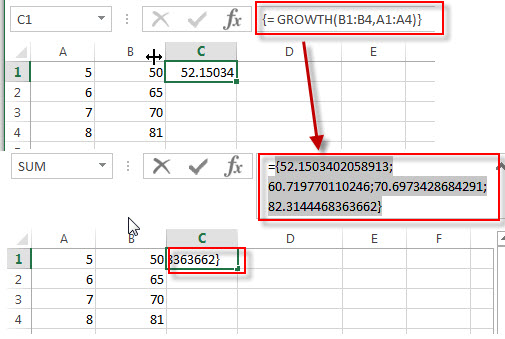# Excel GROWTH Function

This post will guide you how to use the GROWTH function with syntax and examples in Microsoft excel.

### Description

The Excel GROWTH function calculates the predicted exponential growth based on existing data. And it will return the y-values for a sets of new x-values that you specify by using one or more sets of existing x-values and y-values.

The GROWTH function is a build-in function in Microsoft Excel and it is categorized as a Statistical Function.

The GROWTH function is available in Excel 2016, Excel 2013, Excel 2010, Excel 2007, Excel 2003, Excel XP, Excel 2000, Excel 2011 for Mac.

### Syntax

The syntax of the GROWTH function is as below:

`= GROWTH (known_y's, [known_x's], [new_x's], [const])`

Where the GROWTH function arguments are:

• Known_y’s -This is a required argument.  The known y-values that used to predict the exponential growth.
• Known_x’s -This is an optional argument.  The known x-values that used to predict the exponential growth. If this argument is omitted, and it will set as array {1,2,3…} by default.
• New_x’s – This is an optional argument. The new_x’s argument will be used to calculate the corresponding new y-values. If this argument is omitted, it will set as array {1,2,3…} by default.
• Const – This is an optional argument. A logical value specifying whether to force the constant b to equal 1.  And if Const is TRUE, the constant b is calculated normally. If Const is FALSE, the constant b is set to 1.

Note:

• If you want to return array result, then you need to the GROWTH formula as Array formula. When you create array formula, you need to press Ctrl + Shift + Enter on the formula box. And you will see that it will create brackets {} around your formula. You should know that if there is no brackets around your formula, it will not be treated as an array formula.

### Excel GROWTH Function Examples

The below examples will show you how to use Excel GROWTH Function to calculate the predicted exponential growth based on a given set of y-values and one or more sets of x-values.

1# to calculate the predicted exponential growth, with known y-values B1:B4, with known x-values in A1:A4, , using the following array formula (you must be entered as an array formula via pressing Ctrl + Shift + Enter shortcuts):

`{= GROWTH (B1:B4,A1:A4)}`### Related Functions

• Excel FORECAST Function
The Excel FORECAST function used to calculate or predict a future value by using existing values. You can use the FORECAST function to get the predicated y-value for a given x-value. The syntax of the FORECAST function is as below:= FORECAST (x, known_y’s, known_x’s)…